# SSAT Upper Level Math : How to find out if a point is on a line with an equation

## Example Questions

2 Next →

### Example Question #1 : How To Find Out If A Point Is On A Line With An Equation

Solve the following system of equations:

–2x + 3y = 10

2x + 5y = 6

(3, –2)

(–2, –2)

(–2, 2)

(2, 2)

(3, 5)

(–2, 2)

Explanation:

Since we have –2x and +2x in the equations, it makes sense to add the equations together to give 8y = 16 yielding y = 2.  Then we substitute y = 2 into one of the original equations to get x = –2.  So the solution to the system of equations is (–2, 2)

### Example Question #11 : How To Find Out If A Point Is On A Line With An Equation

Which of the following sets of coordinates are on the line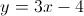?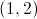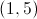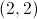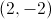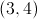Explanation:when plugged in for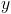andmake the linear equation true, therefore those coordinates fall on that line.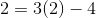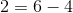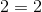Because this equation is true, the point must lie on the line. The other given answer choices do not result in true equalities.

### Example Question #3 : How To Find Out If A Point Is On A Line With An Equation

Which of the following points can be found on the line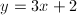?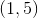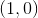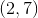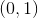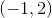Explanation:

We are looking for an ordered pair that makes the given equation true. To solve, plug in the various answer choices to find the true equality.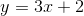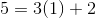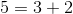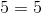Because this equality is true, we can conclude that the pointlies on this line. None of the other given answer options will result in a true equality.

2 Next →

### All SSAT Upper Level Math Resources